# How to Rank Positive and Negative Numbers separately in excel?

The goal of ranking positive and negative numbers separately in Excel can change depending on the details of the task and the context in which it is being used. However, the probable goal is to rank positive and negative numbers separately will help users comprehend how the values in your data collection are distributed. This can assist users in identifying outliers, analyzing trends or patterns, and comprehending the spread and concentration of positive and negative numbers.

## Example 1: To Find Rank Data by Alphabetical Order in Excel

### Step 1

Consider the sample data comprises three columns named student name, Rank Positive, and Rank Negative. Following is a screenshot of this step.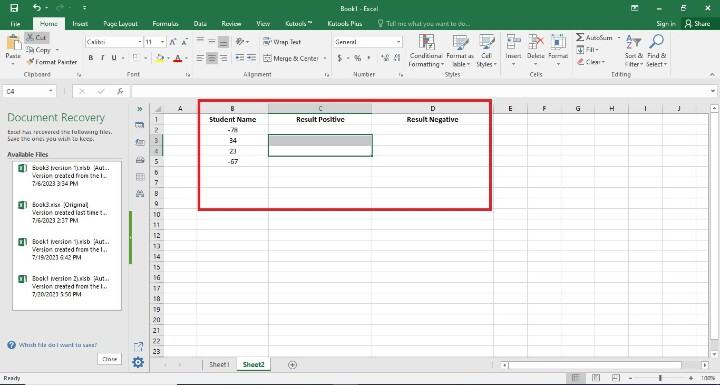### Step 2

In the previous step, the user created the three columns. in this step, the user has entered the formula in the C2 Cell i.e =IF($B2>0,MATCH($B2,SMALL(IF($A$2:$B$5>0,$B$2:$B$5),

ROW(INDIRECT("1:"&COUNTIF($B$2:$B$5,">0")))),0),"") . Following is a screenshot of this step.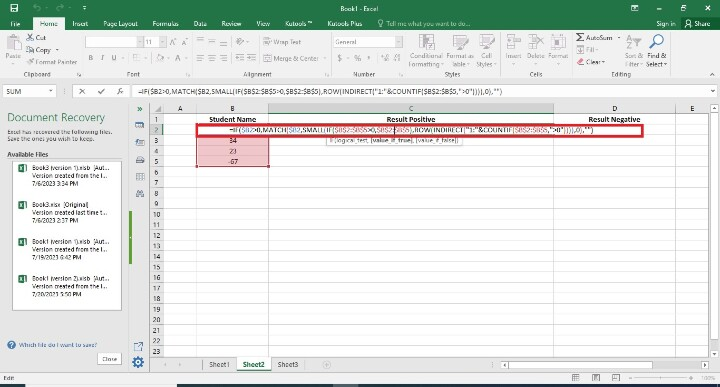## Explanation

=IF($B2>0,MATCH($B2,SMALL(IF($A$2:$B$5>0,$B$2:$B$5),

ROW(INDIRECT("1:"&COUNTIF($B$2:$B$5,">0")))),0),"")

The formula you gave reportedly uses the IF, MATCH, SMALL, IF, ROW, and COUNTIF functions in Excel. Let's deconstruct it

• IF($B2>0, ...) If the value in cell B2 is higher than zero, the statement IF($B2>0,...) is evaluated. If so, the formula continues to calculate. Otherwise, a blank string ("") is returned.

• IF($A$2:$B$5>0, $B$2:$B$5)  Based on a condition, this constructs an array of values from the B column ($B$2:$B$5). The condition determines whether the relevant value ($A$2:$A$5) in column A is greater than 0.

• SMALL(IF($A$2:$B$5>0, $B$2:$B$5), ...)  This returns the array's kth smallest value, which was made in step 2. The ROW(INDIRECT("1:"&COUNTIF($B$2:$B$5,">0"))) component establishes the value for the kth position.

• ROW(INDIRECT("1:"&COUNTIF($B$2:$B$5,">0")))  In column B ($B$2:$B$5), this produces an array of numbers ranging from 1 to the number of positive values. It is applied in step 3 to identify the kth smallest value.

• MATCH(\$B2, SMALL(IF(...)), 0)  This gives the location of the value in cell B2 in the array created in step 3's third step. It determines the value's position among the k smallest values.

### Step 3

The user basically presses the Enter button. In this step, they have seen the blank value in cell C2 because they used the positive formula in the cell. Following is the screenshot of this step.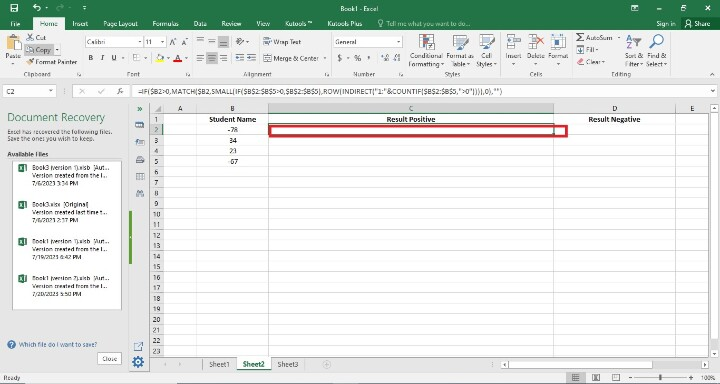### Step 4

Users have to find the remaining Rank Positive result cells. It may be done in two ways. The first way is that users have written the formula in each cell by writing its initial value. The second way is a simple way, where users only drag the fill handle to the final cell. So, In this step, they have seen the rank of positive value only. Following is the screenshot of this step.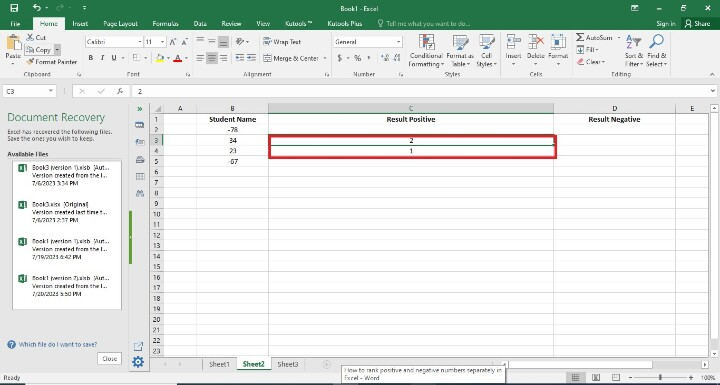### Step 5

The user is writing the formula in the D2 cell. Following is the screenshot of this step.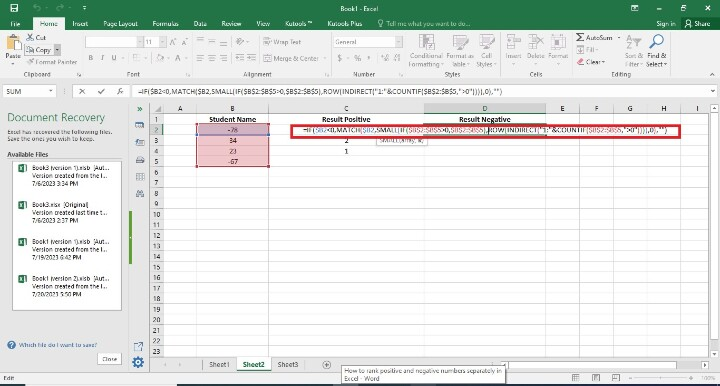### Step 6

The user presses the Enter button. In this step, they have not seen the blank value in cell D2 because they used the negative formula in the cell. Following is the screenshot of this step.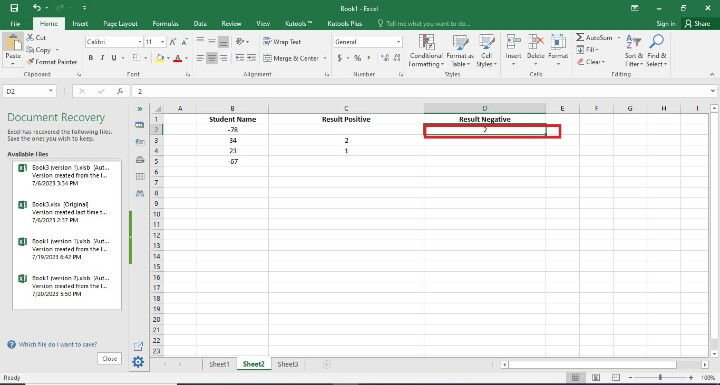### Step 7

In this step, users have to find the remaining Rank Negative result cells. It may be done in two ways. The first way is that users have written the formula in each cell by writing its initial value. The second way is a simple way here users have only drag the fill handle to the final cell. So, In this step, they have seen the rank of negative value only. Following is the screenshot of this step.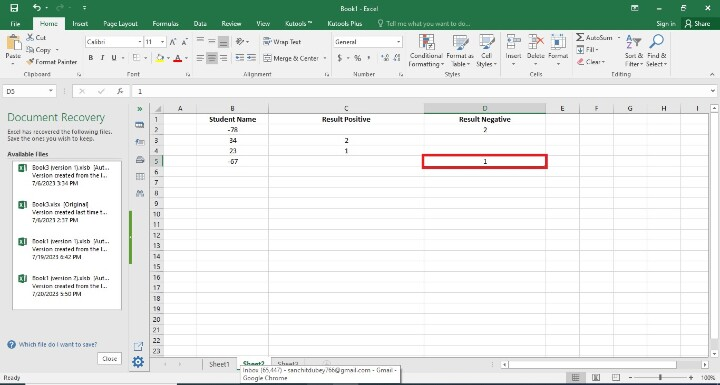## Conclusion

To see the distribution and concentration of values within each category, rank positive and negative numbers separately. You can learn more about the distribution of positive and negative numbers in your data collection and spot any patterns or outliers with the use of this analysis.

The conclusion we reach will ultimately depend on the precise analysis or task at hand. You may better comprehend the distribution, make comparisons, and spot extreme levels within each category by dividing positive and negative integers and ranking them separately in Excel.

Updated on: 21-Aug-2023

64 Views